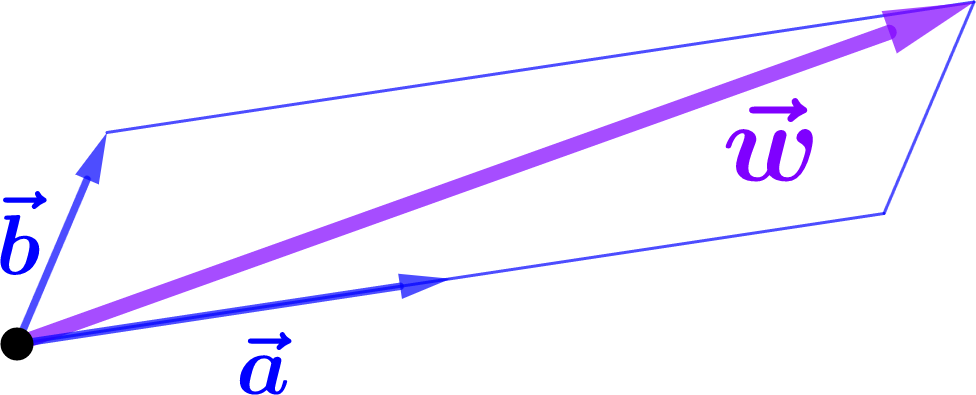A two-dimensional vector, such as the one shown in Figure 1.4.1, can be expressed in terms of how much it goes “to the right” in the direction of $$\aa$$ and how much it goes “up” in the direction of $$\bb,$$ as shown in Figure 1.5.1. This process is called expanding the vector in terms of the basis vectors $$\aa$$ and $$\bb\text{.}$$Figure 1.5.1. A two-dimensional vector $$\ww\text{,}$$ expanded in terms of a basis $$\{\aa,\bb\}\text{.}$$
There is no requirement that the basis vectors $$\aa$$ and $$\bb$$ used in the expansion be “perpendicular”, nor that they have the same “magnitude”. In fact, we do not yet know what those words mean (see Section 1.7)! Nonetheless, we can conclude from Figure 1.5.1 that $$\ww=2\aa+\bb\text{.}$$